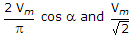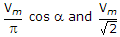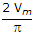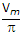# Electronics and Communication Engineering - Power Electronics

### Exercise :: Power Electronics - Section 8

11.

The number of thyristors in a single phase fully controlled bridge rectifier is

 A. 16 B. 8 C. 4 D. 2

Explanation:

No answer description available for this question. Let us discuss.

12.

In a bridge inverter

 A. an output transformer is essential B. an output transformer is not essential C. an output transformer cannot be connected D. an output transformer, if used, can improve the efficiency of the circuit

Explanation:

No answer description available for this question. Let us discuss.

13.

An ideal rectifier should have

 A. 100% efficiency, zero ripple factor, zero harmonic factor and unity displacement factor B. 100% efficiency, zero tipple factor, zero harmonic factor and zero displacement factor C. 100% efficiency, zero ripple factor, unity harmonic factor and unity displacement factor D. 100% efficiency, unity ripple factor, zero harmonic factor and unity displacement factor

Explanation:

No answer description available for this question. Let us discuss.

14.

When thyristors are connected in parallel, the current distribution may become non-uniform due to

 A. inductive effect of current carrying conductors B. capacitive effect of current carrying conductors C. both inductive and capacitive effects D. none of the above

Explanation:

No answer description available for this question. Let us discuss.

15.

A single phase fully controlled bridge converter is fed by a voltage Vm sin ωt. The average and rms values of output voltage are

 A.B.C.cos a and Vm D.cos a and 2 Vm